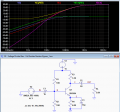# Weird Transient Curve - CE Amplifier Circuit

#### elec_eng_55

Joined May 13, 2018
214
Hi:

I found a CE circuit in a text book and just for fun I created the circuit in LTSpice
(file uploaded). I was wondering why the curve starts at -180mV rather than at
zero.

Thanks,

David

#### Attachments

• 2.2 KB Views: 20

#### ericgibbs

Joined Jan 29, 2010
17,983
hi 55,
Phase shift due to C3 at that frequency.
E

#### elec_eng_55

Joined May 13, 2018
214
hi 55,
Phase shift due to C3 at that frequency.
E
Hi Eric:

I am still learning with a long way to go. Does that mean that the value of C3 should
be changed?

David

#### ericgibbs

Joined Jan 29, 2010
17,983
hi 55,
Have you done an AC analysis, that will reveal the frequency response.?
Try changing C3 values.
E

#### crutschow

Joined Mar 14, 2008
32,849
Does that mean that the value of C3 should
be changed?
Yes, it should be increased if you want flat response to a lower frquency.

Note that the rolloff point for that capacitor (frequency where the gain starts to drop) is mainly determined by the equivalent emitter output impedance at its operating point (about 2.5Ω for this circuit), not the value of R4.
This is a common error when determining the common-emitter stage frequency response.

The frequency where the capacitive impedance equals R4 is the point where the transistor stage gain rises 3dB above its minimum value of ≈ R3/R4 (-6.3dB).

#### elec_eng_55

Joined May 13, 2018
214
hi 55,
Have you done an AC analysis, that will reveal the frequency response.?
Try changing C3 values.
E
Thanks.
I haven't tried this frequency response curve before. I remember a frequency response
curve from high school but the vertical axis was output voltage and the horizontal axis
was frequency. This is not familiar to me.

I did increase C3 value and it cleared up the issue.

I would like to understand this response curve though.

David

#### elec_eng_55

Joined May 13, 2018
214
Yes, it should be increased if you want flat response to a lower frquency.

Note that the rolloff point for that capacitor (frequency where the gain starts to drop) is mainly determined by the equivalent emitter output impedance at its operating point (about 2.5Ω for this circuit), not the value of R4.
This is a common error when determining the common-emitter stage frequency response.

The frequency where the capacitive impedance equals R4 is the point where the transistor stage gain rises 3dB above its minimum value of ≈ R3/R4 (-6.3dB).
Thanks crutschow.

Joined Mar 10, 2018
4,057
Sure its not the phase shift caused by the zero of C1 and Zin ? Actually it is
the effects or C1, C2, C3 and associated Re(Z) and the fact the transistor
has finite GBW. As to which causes the most phase shift the analysis would be
done node by node with the sim.

Or use signal flow graph coupled with PFE to get at each elemental contributor.

This plot is blue output, green base of transistor.

Regards, Dana.

#### elec_eng_55

Joined May 13, 2018
214
Sure its not the phase shift caused by the zero of C1 and Zin ? Actually it is
the effects or C1, C2, C3 and associated Re(Z) and the fact the transistor
has finite GBW. As to which causes the most phase shift the analysis would be
done node by node with the sim.

Or use signal flow graph coupled with PFE to get at each elemental contributor.

This plot is blue output, green base of transistor.

Thanks Dana.

Regards, Dana.

#### crutschow

Joined Mar 14, 2008
32,849
I would like to understand this response curve though.
Here are the response curves for various points in the circuit.
Note that an AC voltage of 1V is defined as 0dB for the plots so that's what the input was set to.
(The AC analysis uses linear models, so the input can be any voltage even though in real life. or in the transient analysis, that would saturate the amplifier).

V(b) is the base voltage, whose low frequency rolloff is caused by C1.

V(C)/V(B) is the gain between the base and collector, whose low frequency rolloff is caused by C3.

V(c) is the collector voltage, whose rolloff is the sum of the rolloffs from C1 and C3.

V(out)/V(C) is the difference in voltage between the collector and the output, whose rolloff is determined by C2 (increased to 2μF to differentiate its rolloff from V(b).

V(out) is the output voltage, whose rolloff is the sum of the rolloffs from C1, C3, and C2.Joined Mar 10, 2018
4,057
Phase response of various circuit nodes, attached.

Regards, Dana

#### elec_eng_55

Joined May 13, 2018
214
Phase response of various circuit nodes, attached.

Regards, Dana
That's great. Thanks so much Dana.

David

#### elec_eng_55

Joined May 13, 2018
214
Here are the response curves for various points in the circuit.
Note that an AC voltage of 1V is defined as 0dB for the plots so that's what the input was set to.
(The AC analysis uses linear models, so the input can be any voltage even though in real life. or in the transient analysis, that would saturate the amplifier).

V(b) is the base voltage, whose low frequency rolloff is caused by C1.

V(C)/V(B) is the gain between the base and collector, whose low frequency rolloff is caused by C3.

V(c) is the collector voltage, whose rolloff is the sum of the rolloffs from C1 and C3.

V(out)/V(C) is the difference in voltage between the collector and the output, whose rolloff is determined by C2 (increased to 2μF to differentiate its rolloff from V(b).

V(out) is the output voltage, whose rolloff is the sum of the rolloffs from C1, C3, and C2.